# NPTEL The Joy of Computing using Python Assignment 2 Answers 2023

NPTEL The Joy of Computing using Python Assignment 2 Answers 2023:- All the Answers provided below to help the students as a reference, You must submit your assignment at your own knowledge.

## NPTEL The Joy Of Computing Using Python Week 2 Assignment Answer 2023

1. Which of the following is a high-level programming language?

• Assembly
• CPython
• Machine
• Language
`Answer :- Click Here`

2. Which of the following is an example of a front-end programming language?

• PHP
• Java
• HTML
• SQL
`Answer :- Click Here`

3. Which of the following is used to store data in a programming language?

• Variables
• Arrays
• Loops
• Conditionals
`Answer :- Click Here`

4. Which statement will print ‘The joy of computing’?

• print(The joy of computing)
• print The joy of computing
• printf(‘The joy of computing)
• print(‘The joy of computing’)
`Answer :- Click Here`

5. What should be the value of _ to print all numbers from 0-10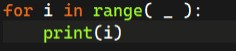• 10
• 9
• 11
• None of the above
`Answer :- Click Here`

6. Which of the following keyword is used to declare a function in a programming language?

• def
• var
• int
• float
`Answer :- Click Here`

7. What will be the output of the following code?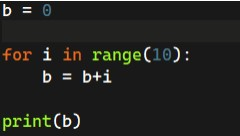• 0
• 45
• 43
• 50
`Answer :- Click Here`

8. What value will c store in it after the execution of the below code?
c=b**3

• Value of b multiplied by 3
• Cube of b
• Value of b multiplied with 3 twice
• It will throw an error
`Answer :- Click Here`

9. What will be the output? suppose the input is 30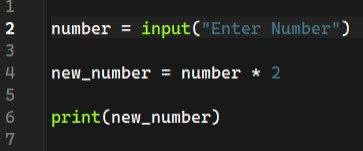• 60
• Error
• 3030
• 30
`Answer :- Click Here`

10. What is the output for the following program?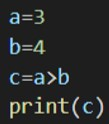• True
• False
• It will display an error message
• Boolean
`Answer :- Click Here `

## NPTEL The Joy of Computing using Python Assignment 2 Answers [July 2022]

1. What is the correct command to show the value stored in a variable?

a. show()
b. cout()
c. print()
d. out()

`Answer:- c`

2. What will be the output of the following piece of code?

a. Hello Mr. Bond 34
b. Hello Mr. 34
c. Hello Mr. Bond 35
d. Hello Mr. Bond 34

`Answer:- c `

3. What will be the output of the following code?

a. 10,10
b. 10
c. No output
d. Error

`Answer:- d`

4. What is the extension of a python file?

a. sc
b. py
c. md
d. Cp

`Answer:- b`

5. what will be the output of the following code, if the given input is 5?

A = input()
print(A*5)

a. 25
b. 55555
c. 55
d. 5

`Answer:- b `

6. Which of the following commands are correct to take input from a user?

a. n = input(‘Hello’)
b. n = input(“42”)
c. n = input(‘42’)
d. n = input(Hello)
e. n = input()

`Answer:- a, b, c, e`

7. What will be the output of the following code if the input is ‘5’?

a. 25
b. 25.0
c. Error
d. 5555

`Answer:- a`

8. Which of the following blocks of codes are correct?(Assume x and y are initialized)

`Answer:- b`

9. Which of the following statements are correct regarding the following code?

for i in range(a):

a. i will take values from 0 to a.
b. i will take values from 1 to a.
c. i will take values from 0 to a-1.
d. i will take values from 1 to a-1.

`Answer:- For Answer Click Here`

10. What will be the output of the following code?

a. 1,2,3,4,5,6,7,8,9,10
b. 2,4,6,8,10
c. 0,1,2,3,4,5,6,7,8,9,10
d. Error

`Answer:- b`

## NPTEL The Joy of Computing using Python Assignment 2 Programming Solutions:-

Q1. Write a program to take an input from the user and print that input.

Program:-

``````string = input()
print(string, end="")``````

Q2. Write a program to take an input of two numbers A, and B and print the difference A-B

Program:-

``````A = int(input())
B = int(input())

z = A-B

print(z, end="")``````

Q3. Take a string S and an integer A as an input from a user. Write a program to print string S, A number of times.

Program:-

``````S = input()
A = int(input())

for i in range(1,A+1):
print(S)``````

## What is The Joy Of Computing Using Python?

The Joy Of Computing Using Python is a fun-filled whirlwind tour of 30 hrs, covering everything you need to know to fall in love with the most sought-after skill of the 21st century. This course includes examples of analytics in a wide variety of industries, and we hope that students will learn how you can use analytics in their careers and life. One of the most important aspects of this course is that you, the student, are getting hands-on experience creating analytics models; we, the course team, urge you to participate in the discussion forums and to use all the tools available to you while you are in the course!

CRITERIA TO GET A CERTIFICATE

Average assignment score = 25% of the average of best 8 assignments out of the total 12 assignments given in the course.
Exam score = 75% of the proctored certification exam score out of 100

Final score = Average assignment score + Exam score

YOU WILL BE ELIGIBLE FOR A CERTIFICATE ONLY IF THE AVERAGE ASSIGNMENT SCORE >=10/25 AND EXAM SCORE >= 30/75. If one of the 2 criteria is not met, you will not get the certificate even if the Final score >= 40/100.

## NPTEL The Joy of Computing using Python Assignment 2 Answers [Jan 2022]

Q1. Which statement will print ‘The joy of computing’?

(A) print(The joy of computing)
(B) print The joy of computing printf
(C) (‘The joy of computing’)
(D) print(‘The joy of computing’)

Answer:- (D) print(‘The joy of computing’)

Q2. What is the output of the following code?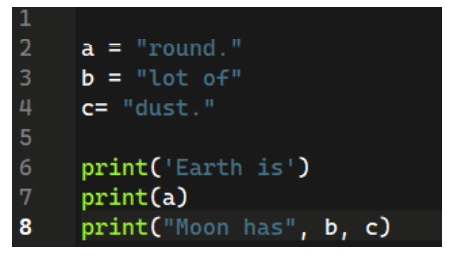(A) Earth is round.
Moon has lot of dust.
(B) Earth is
round.
Moon has
lot of dust.
(C) Earth is
round.
Moon has lot of dust
(D) Earth is round.Moon has lot of dust.

Q3. What should be the syntax of getting age as an input from a user in python?

(A) Age = int(“Enter Age”)
(B) Age = input(“Enter Age”)
(C) Age = get(“Enter Age”)
(D) Age = input(Enter Age)

Q4. What should be the value of _ to print all numbers from 0-10?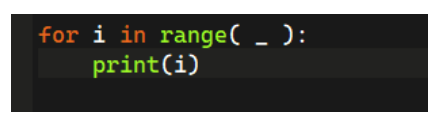(A) 10
(B) 9
(C) 11
(D) None of the above

Q5. What will be the output of the following code?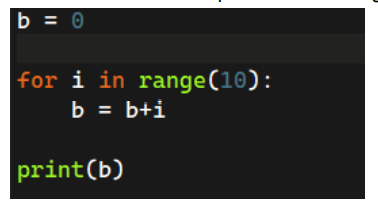(A) 0
(B) 45
(C) 43
(D) 50

Q6. What will be the output? suppose the input is 20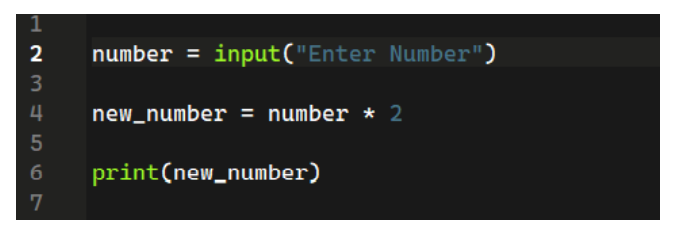(A) 40
(B) Error
(C) 2020
(D) None of the above

Q7. What will be the output of the following code be?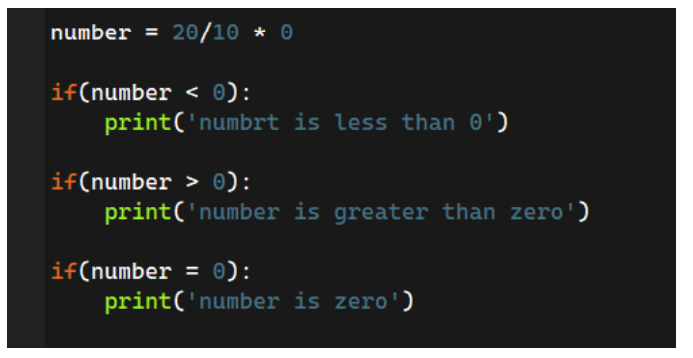(A) number is less than 0
(B) number is greater than 0
(C) number is zero
(D) Error

Q8. What will be the output of the following code?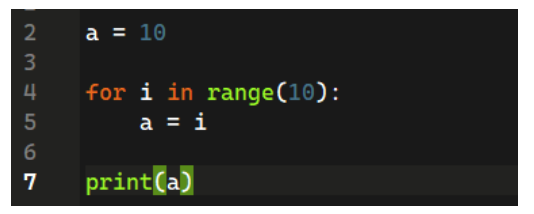(A) 9
(B) 10
(C) 0
(D) None of the above

Q9. What will be the output of the following code?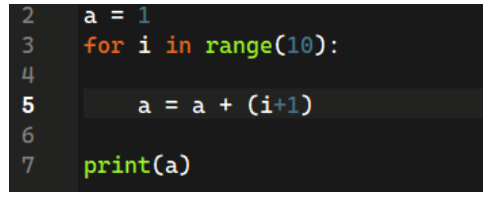(A) 56
(B) 45
(C) 0
(D) Error

Q10. What will be the output of the following code?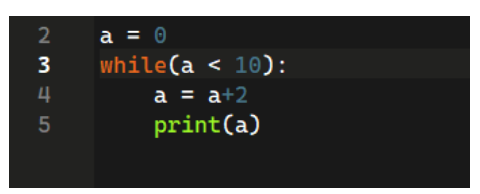(A) 1,2,3,4,5,6,7,8,9,10
(B) 2,4,6,8,10
(C) 0,1,2,3,4,5,6,7,8,9,10
(D) Error